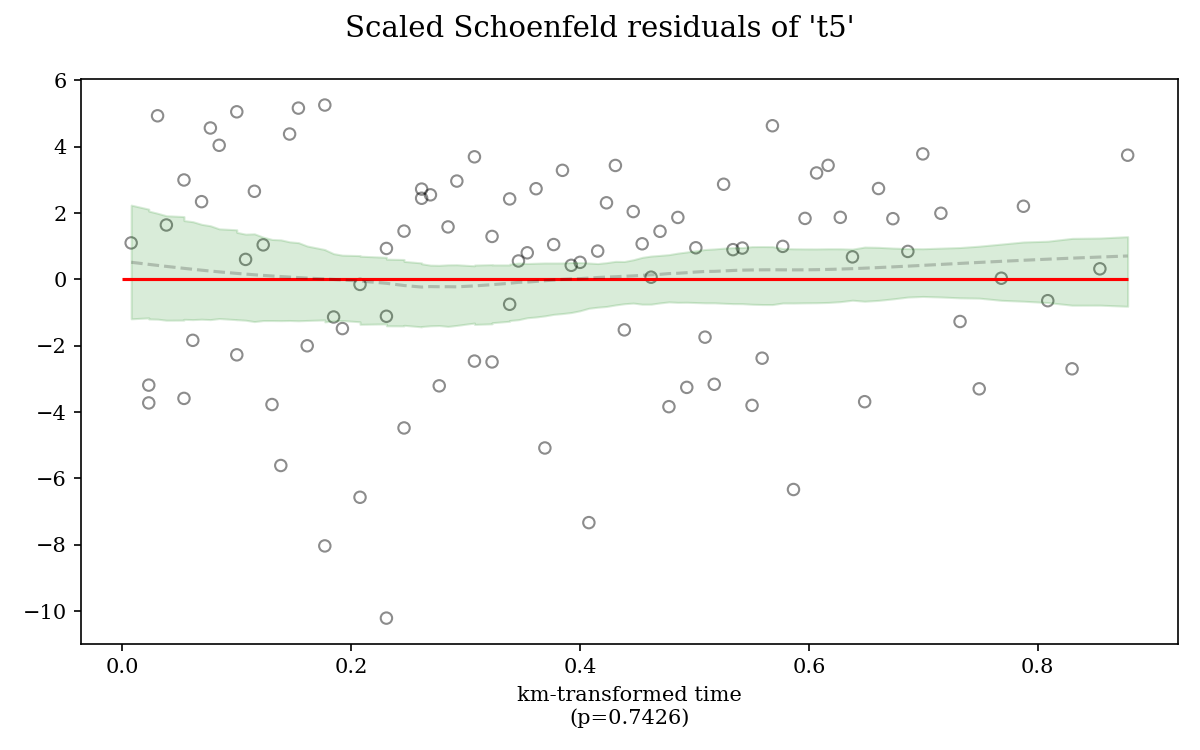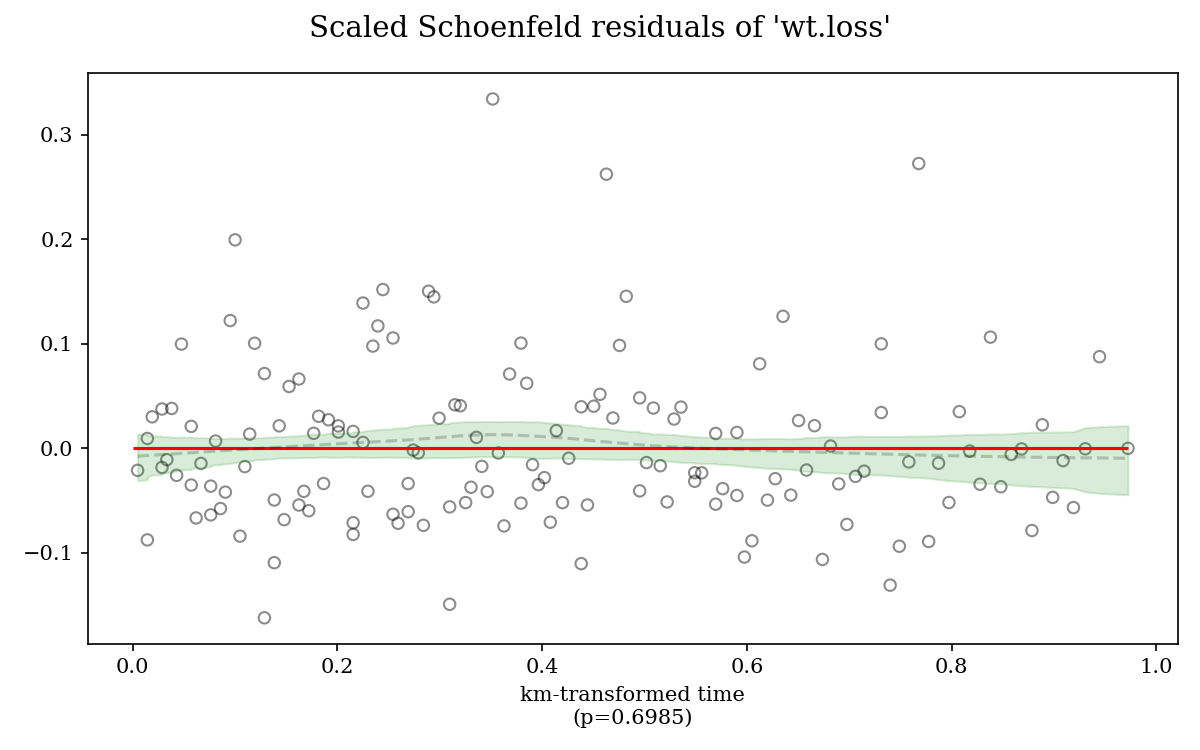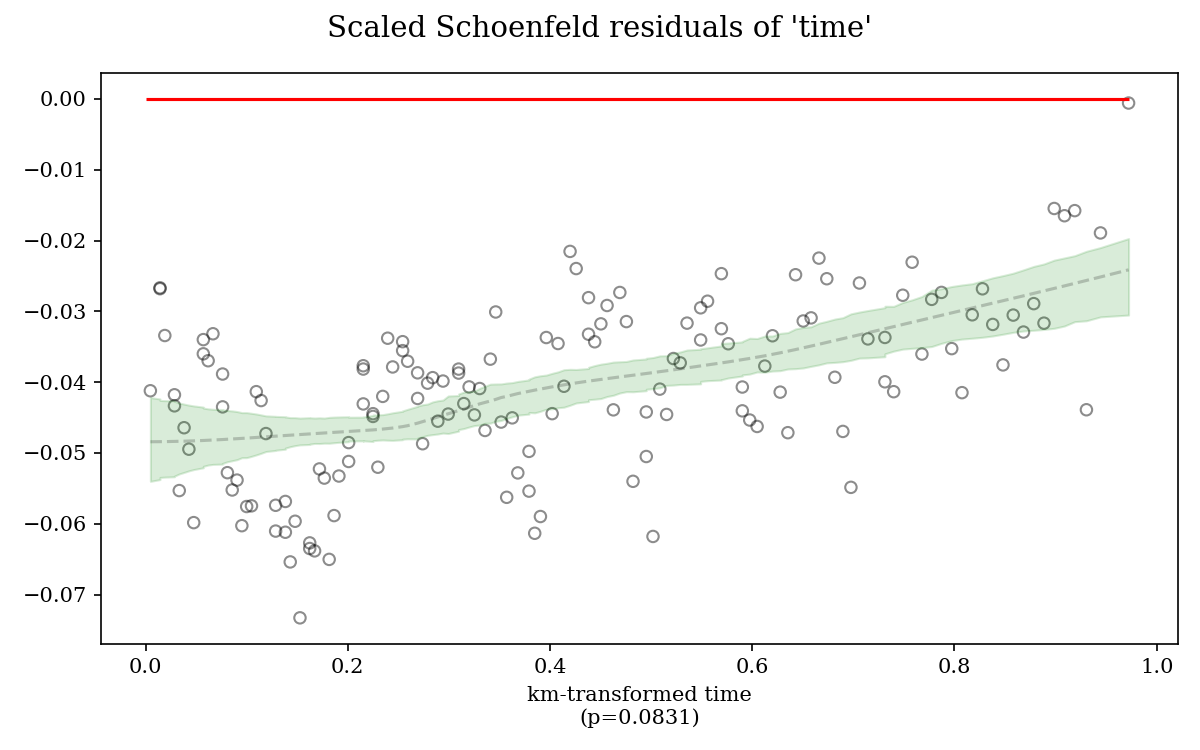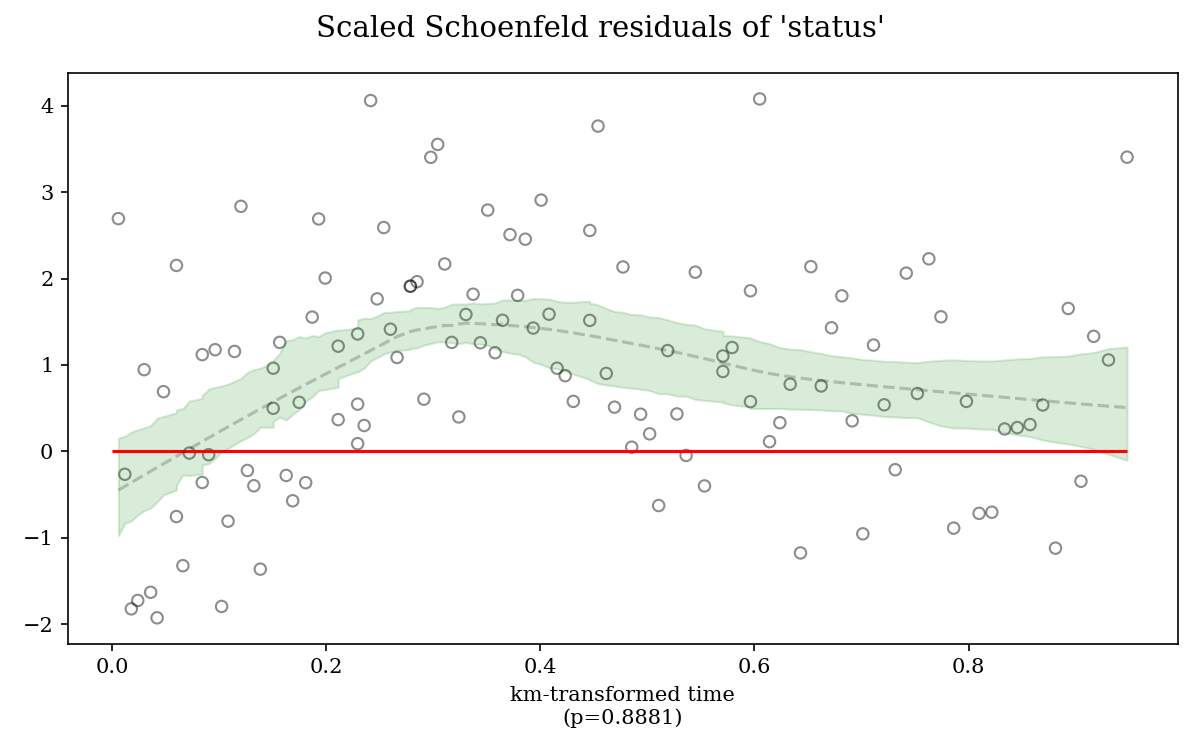# Proportional Hazards assessment in Cox regression analysis

Modified on Fri, 09 Jul 2021 at 04:40 PM

The proportional hazards is the backbone assumption of a Cox model. It suggests that all patients of a study have the same hazard function, but a unique scaling factor in front. This is equivalent to having the ratio of the hazards for any two individuals constant over time and thus proportional. In the Cox model regression, this implies that the effect of a given covariate does not change over time.

To check proportional hazards assumption we rely on visual plots of the “Scaled Schoenfeld Residuals” over which we plot a “lowess” curve with a confidence zone.

Assessing the proportional hazards is so reduced to answering the same easy question: Is the red line within the green zone ?

The answer should be yes when the red line is within the green area over 95% of the time. If not, the assumption is violated and the current solution is to completely delete the variable (Other solutions for this problem will be provided by our software in new versions to come).

Typical valid plots of the proportional hazards assumption looks like the ones below:For the violation of the proportional hazards assumption, examples are shown below: